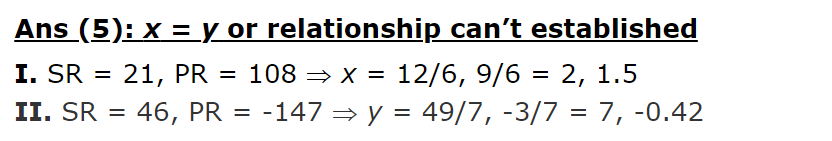# Quadratic Equation Set- 2 For SBI PO Exam 2023

Quadratic equation Quiz for Bank pre exam : Quadratic equation is an important chapter of quantitative aptitude in competitive exams such as IBPS, SBI and RBI PO & Clerk. Solving more and more Quadratic equation Questions will give you a good command on this topic. So, here we are giving you Quadratic equation Questions and answers for IBPS, SBI and RBI PO & Clerk exam. This Quadratic equation Quiz contains questions based on the latest exam pattern of IBPS, SBI and RBI PO & Clerk exam. Here, answers and solution are also provided in this Quadratic equation quiz. Attempt this Quadratic equation Quiz and practice from it to master this topic.
Quadratic Equation Quiz to improve your Quantitative Aptitude for SBI Po & SBI clerk exam IBPS PO Reasoning , IBPS Clerk Reasoning , IBPS RRB Reasoning, LIC AAO ,LIC Assistant and other competitive exam

1➤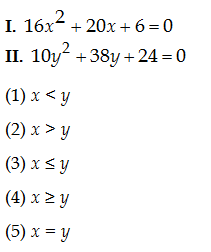=>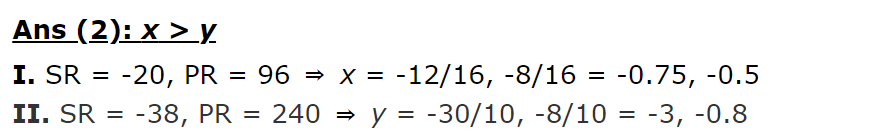2➤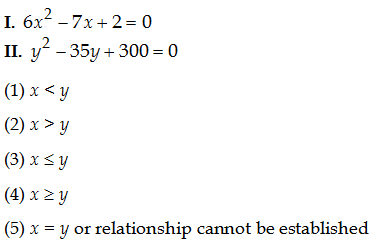=>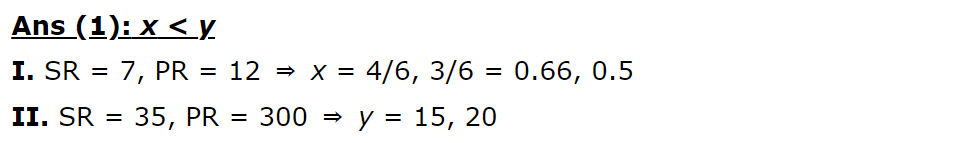3➤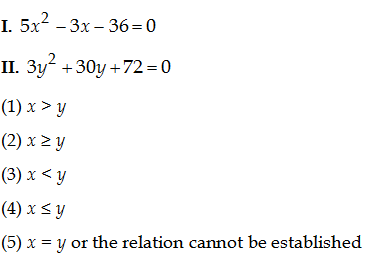=>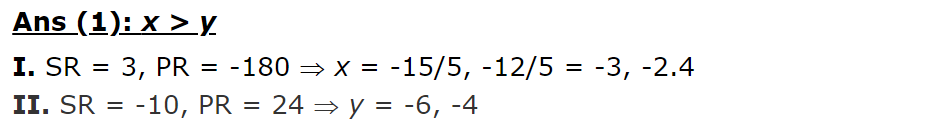4➤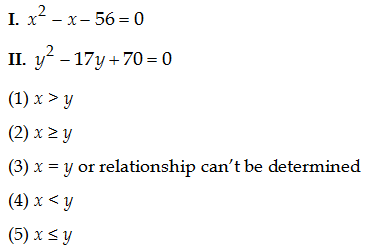=>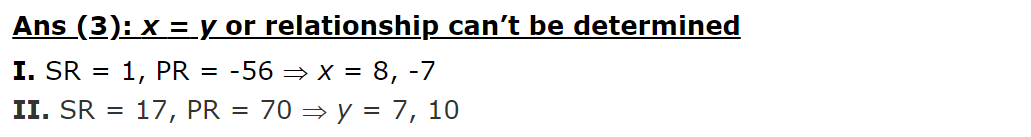5➤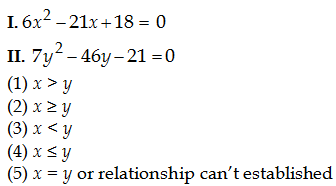=>